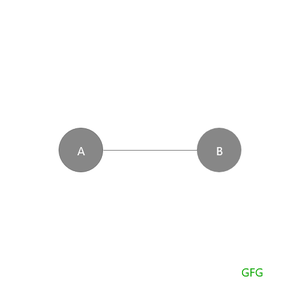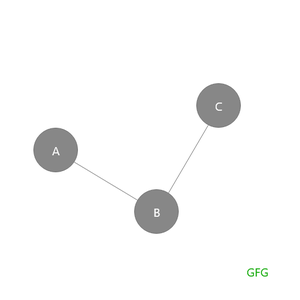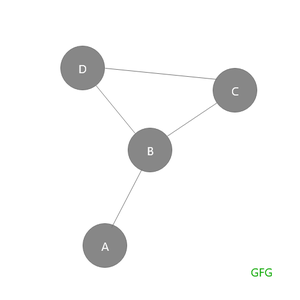Open in App
Not now

# Pendant Vertices, Non-Pendant Vertices, Pendant Edges and Non-Pendant Edges in Graph

• Last Updated : 12 Jan, 2023

Pre-requisites: Handshaking theorem.

### Pendant Vertices

Let G be a graph, A vertex v of G is called a pendant vertex if and only if v has degree 1. In other words, pendant vertices are the vertices that have degree 1, also called pendant vertex

Note: Degree = number of edges connected to a vertex

In the case of trees, a pendant vertex is known as a terminal node or leaf node, or leaf since it has only 1 degree. Remember leaf nodes have only 1 degree so pendant vertex is called a leaf node in the case of trees.

Example: In the given diagram A and B are pendant vertices because each of them has degree 1.Graph 1.0

Let’s take this example to print all the pendant vertices in the graph.

## C++

 `// C++ program for the above approach` `#include ` `using` `namespace` `std;`   `// Function to print all the pendant` `// vertices` `void` `printPendantVertices(map<``char``, vector<``char``> > graph)` `{`   `    ``// All the vectors which contain only 1` `    ``// vertex i.e, size 1 has only 1 edge` `    ``// hence a pendant vertex.` `    ``for` `(``auto` `x : graph) {` `        ``if` `(x.second.size() == 1) {` `            ``cout << x.first << ``" "``;` `        ``}` `    ``}` `}`   `// Driver Code` `int` `main()` `{`   `    ``map<``char``, vector<``char``> > graph;` `    ``graph[``'A'``].push_back(``'B'``);` `    ``graph[``'B'``].push_back(``'A'``);` `    ``graph[``'C'``].push_back(``'B'``);` `    ``graph[``'B'``].push_back(``'C'``);`   `    ``printPendantVertices(graph);`   `    ``return` `0;` `}`

## Java

 `// Java program for the above approach` `import` `java.util.*;`   `class` `GFG ` `{`   `  ``// Function to print all the pendant` `  ``// vertices` `  ``static` `void` `printPendantVertices(` `    ``HashMap > graph)` `  ``{`   `    ``// All the vectors which contain only 1` `    ``// vertex i.e, size 1 has only 1 edge` `    ``// hence a pendant vertex.` `    ``for` `(Character x : graph.keySet()) {` `      ``if` `(graph.get(x).size() == ``1``) {` `        ``System.out.print(x + ``" "``);` `      ``}` `    ``}` `  ``}`   `  ``public` `static` `void` `main(String[] args)` `  ``{` `    ``HashMap > graph` `      ``= ``new` `HashMap<>();` `    ``graph.put(``'A'``, ``new` `ArrayList<>());` `    ``graph.get(``'A'``).add(``'B'``);` `    ``graph.put(``'B'``, ``new` `ArrayList<>());` `    ``graph.get(``'B'``).add(``'A'``);` `    ``graph.put(``'C'``, ``new` `ArrayList<>());` `    ``graph.get(``'C'``).add(``'B'``);` `    ``graph.get(``'B'``).add(``'C'``);`   `    ``printPendantVertices(graph);` `  ``}` `}`   `// This code is contributed by Karandeep1234`

## Python3

 `# Python program for the above approach`   `# Function to print all the pendant` `# vertices` `def` `printPendantVertices(graph):` `  `  `# All the vectors which contain only 1` `# vertex i.e, size 1 has only 1 edge` `# hence a pendant vertex.` `    ``for` `x,y ``in` `graph.items():` `        ``if` `(``len``(y) ``=``=` `1``):` `            ``print``(x, end ``=` `" "``)`   `# Driver Code`   `graph ``=``{}` `graph[``"A"``] ``=`  `[``"B"``]` `graph[``"B"``] ``=` `[``"A"``]` `graph[``"C"``] ``=` `[``"B"``]` `graph[``"B"``].append(``"A"``)`   `printPendantVertices(graph)`   `# This code is contributed by shinjanpatra`

## C#

 `using` `System;` `using` `System.Collections.Generic;`   `class` `GFG` `{` `  `  `  ``// Function to print all the pendant vertices` `  ``static` `void` `printPendantVertices(` `    ``Dictionary<``char``, List<``char``> > graph)` `  ``{` `    `  `    ``// All the vectors which contain only 1` `    ``// vertex i.e, size 1 has only 1 edge` `    ``// hence a pendant vertex.` `    ``foreach``(``var` `x ``in` `graph.Keys)` `    ``{` `      ``if` `(graph[x].Count == 1) {` `        ``Console.Write(x + ``" "``);` `      ``}` `    ``}` `  ``}`   `  ``public` `static` `void` `Main(``string``[] args)` `  ``{` `    ``var` `graph = ``new` `Dictionary<``char``, List<``char``> >();` `    ``graph[``'A'``] = ``new` `List<``char``>();` `    ``graph[``'A'``].Add(``'B'``);` `    ``graph[``'B'``] = ``new` `List<``char``>();` `    ``graph[``'B'``].Add(``'A'``);` `    ``graph[``'C'``] = ``new` `List<``char``>();` `    ``graph[``'C'``].Add(``'B'``);` `    ``graph[``'B'``].Add(``'C'``);`   `    ``printPendantVertices(graph);` `  ``}` `}`   `// This code is contributed by ishankhandelwals.`

## Javascript

 ``

Output

`A C `

### Non-Pendant Vertices

Non-Pendant Vertices are the vertices that have degrees other than 1. In the case of trees, a non-pendant vertex is a non-leaf node, since it does not have degree 1 ( leaf nodes have degree 1).

Example: In the given diagram A and C are pendant vertices because A and C have degree 1, B is a non-pendant vertex because it contains degrees other than 1 which is 2.Graph 2.0

### Pendant Edge

An edge of a graph is said to be a pendant edge if and only if one of its vertices is a pendant vertex.

Example: In the given diagram AB is a pendant edge since it contains pendant vertex as one of its vertexes.Graph 3.0

### Non-Pendant Edge

An edge of a graph is said to be a non-pendant edge if it does not contain a pendant vertex as one of its vertexes.

Example: in the given diagram AB is a pendant edge since it has pendant vertex (A) as one of its vertexes. BD, BC, DC are non-pendant vertices.Graph 4.0

### Examples:

Let us see some questions based examples on the above topics:

Q1. Suppose that a tree T has 2 vertices of degree 2, 4 vertices of degree 3, and 3 vertices of degree 4. find the number of pendant vertices in T.

Finding number pendant vertices is nothing but finding the number of leaf nodes.
Let’s use the Handshaking Theorem formula

Sum of all degrees  = 2 * (Sum of Edges)

(2 vertices) * (2 degrees) + (4 vertices) * (3 degrees) + (3 vertices) * (4 degrees) + (k vertices) * (1 degree) = (2 * edges)

where
k is pendant vertices or leaf nodes which have degree 1
e is total number of edges in the tree

2*2 + 4*3 + 3*4 + k*1 = 2*e      ——-(1)

remember : number of edges = number of vertices – 1

e=(2+4+3+k)-1

e=9+k-1

e=8+k                                         ——-(2)

putting equation 2 in 1 gives

4 + 12 + 12 + k = 2(8+k)

28 + k = 16 + 2k

-2k + k = 16 – 28

-k = -12

k = 12

so total number of pendant vertices are 12

Q2. If a tree T has 4 vertices of degree 2, 1 vertex of degree 3 and 2 vertices of degree 4 and 1 vertex of degree 5. find the number of pendant vertices in T.

Finding number pendant vertices is nothing but finding the number of leaf nodes.

Let’s use the Handshaking Theorem formula

Sum of all degrees  = 2 * (Sum of Edges)

(4 vertices)*(2 degrees) + (1 vertex)*(3 degrees) + (2 vertices)*(4 degrees) + (1 vertex)*(5 degree) + (k vertices)*(1 degree) = (2 * edges)

where
k is pendant vertices or leaf nodes which have degree 1
e is total number of edges in the tree

4*2 + 1*3 + 2*4 + 1*5 + k*1 = 2*e      ——-(1)

remember number of edges = number of vertices – 1

e=(4+1+2+1+k)-1

e=8+k-1

e=7+k                                         ——-(2)

putting equation 2 in 1 gives

8 + 3 + 8 + 5 + k = 2(7+k)

24 + k = 14 + 2k

-2k + k = 14 – 24

-k = -10

k = 10

so total number of pendant vertices are 10

My Personal Notes arrow_drop_up
Related Articles